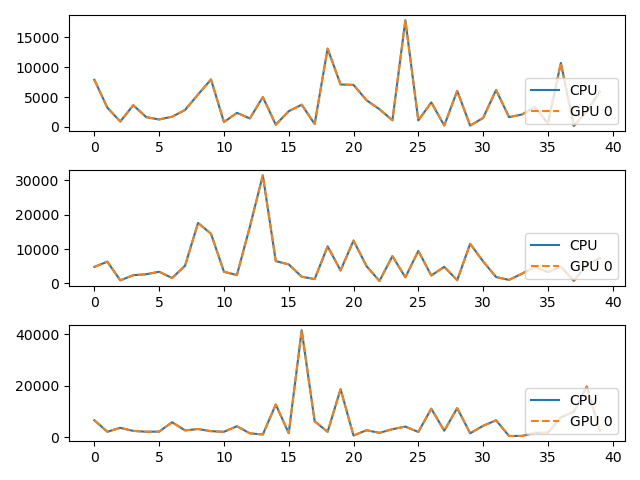GPU Selection¶

On multi-device clusters, let’s see how to select the card on which a KeOps operation will be performed.

Setup¶

Standard imports:

import matplotlib.pyplot as plt
import numpy as np

from pykeops.numpy import Genred, Vi, Vj
from pykeops.common.gpu_utils import get_gpu_number
import pykeops.config

Define the list of gpu ids to be tested:

# By default we assume that there are two GPUs available with 0 and 1 labels:
gpuids = [0, 1] if get_gpu_number() > 1 else 

KeOps Kernel using Genred¶

Define some arbitrary KeOps routine:

formula = "Square(p-a) * Exp(x+y)"
variables = ["x = Vi(3)", "y = Vj(3)", "a = Vj(1)", "p = Pm(1)"]

dtype = "float32"  # May be 'float32' or 'float64'

my_routine = Genred(formula, variables, reduction_op="Sum", axis=1, dtype=dtype)

Generate some data, stored on the CPU (host) memory:

M = 1000
N = 2000
x = np.random.randn(M, 3).astype(dtype)
y = np.random.randn(N, 3).astype(dtype)
a = np.random.randn(N, 1).astype(dtype)
p = np.random.randn(1).astype(dtype)

Launch our routine on the CPU:

c = my_routine(x, y, a, p, backend="CPU")

And on our GPUs, with copies between the Host and Device memories:

if pykeops.config.gpu_available:
for gpuid in gpuids:
d = my_routine(x, y, a, p, backend="GPU", device_id=gpuid)
print(
"Relative error on gpu {}: {:1.3e}".format(
gpuid, float(np.sum(np.abs(c - d)) / np.sum(np.abs(c)))
)
)

# Plot the results next to each other:
for i in range(3):
plt.subplot(3, 1, i + 1)
plt.plot(c[:40, i], "-", label="CPU")
plt.plot(d[:40, i], "--", label="GPU {}".format(gpuid))
plt.legend(loc="lower right")

plt.tight_layout()
plt.show()Out:

Relative error on gpu 0: 5.472e-07
Relative error on gpu 1: 5.472e-07
/data/jean/keops/pykeops/examples/numpy/plot_gpu_select_numpy.py:77: MatplotlibDeprecationWarning: Adding an axes using the same arguments as a previous axes currently reuses the earlier instance.  In a future version, a new instance will always be created and returned.  Meanwhile, this warning can be suppressed, and the future behavior ensured, by passing a unique label to each axes instance.
plt.subplot(3, 1, i + 1)
/data/jean/keops/pykeops/examples/numpy/plot_gpu_select_numpy.py:77: MatplotlibDeprecationWarning: Adding an axes using the same arguments as a previous axes currently reuses the earlier instance.  In a future version, a new instance will always be created and returned.  Meanwhile, this warning can be suppressed, and the future behavior ensured, by passing a unique label to each axes instance.
plt.subplot(3, 1, i + 1)
/data/jean/keops/pykeops/examples/numpy/plot_gpu_select_numpy.py:77: MatplotlibDeprecationWarning: Adding an axes using the same arguments as a previous axes currently reuses the earlier instance.  In a future version, a new instance will always be created and returned.  Meanwhile, this warning can be suppressed, and the future behavior ensured, by passing a unique label to each axes instance.
plt.subplot(3, 1, i + 1)

Using LazyTensor¶

Launch our routine on the CPU:

xi, yj, aj = Vi(x), Vj(y), Vj(a)
c = ((p - aj) ** 2 * (xi + yj).exp()).sum(axis=1, backend="CPU")

And on the GPUs, with copies between the Host and Device memories:

if pykeops.config.gpu_available:
for gpuid in gpuids:
d = ((p - aj) ** 2 * (xi + yj).exp()).sum(
axis=1, backend="GPU", device_id=gpuid
)
print(
"Relative error on gpu {}: {:1.3e}".format(
gpuid, float(np.sum(np.abs(c - d)) / np.sum(np.abs(c)))
)
)

# Plot the results next to each other:
for i in range(3):
plt.subplot(3, 1, i + 1)
plt.plot(c[:40, i], "-", label="CPU")
plt.plot(d[:40, i], "--", label="GPU {}".format(gpuid))
plt.legend(loc="lower right")

plt.tight_layout()
plt.show()Out:

Relative error on gpu 0: 5.472e-07
Relative error on gpu 1: 5.472e-07
/data/jean/keops/pykeops/examples/numpy/plot_gpu_select_numpy.py:111: MatplotlibDeprecationWarning: Adding an axes using the same arguments as a previous axes currently reuses the earlier instance.  In a future version, a new instance will always be created and returned.  Meanwhile, this warning can be suppressed, and the future behavior ensured, by passing a unique label to each axes instance.
plt.subplot(3, 1, i + 1)
/data/jean/keops/pykeops/examples/numpy/plot_gpu_select_numpy.py:111: MatplotlibDeprecationWarning: Adding an axes using the same arguments as a previous axes currently reuses the earlier instance.  In a future version, a new instance will always be created and returned.  Meanwhile, this warning can be suppressed, and the future behavior ensured, by passing a unique label to each axes instance.
plt.subplot(3, 1, i + 1)
/data/jean/keops/pykeops/examples/numpy/plot_gpu_select_numpy.py:111: MatplotlibDeprecationWarning: Adding an axes using the same arguments as a previous axes currently reuses the earlier instance.  In a future version, a new instance will always be created and returned.  Meanwhile, this warning can be suppressed, and the future behavior ensured, by passing a unique label to each axes instance.
plt.subplot(3, 1, i + 1)

Total running time of the script: ( 0 minutes 0.720 seconds)

Gallery generated by Sphinx-Gallery